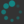## Problems

In this problem set, we will use angle brackets to denote points, e.g. $$\pt10,$$ and square brackets to denote vectors, e.g. $\vec32.$ This is not standard notation. Typically both are denoted by square brackets (or parentheses), and distinguished by context (if at all).

1. We reduced the problem of computing $$\RotP\theta AB$$ to the problem of computing $$\RotVparen\theta {B-A},$$ and found a formula for that, but we never explicitly wrote down the resulting formula for $$\RotP\theta AB.$$ So suppose that $$A = \pt {a_1}{a_2}$$ and $$B = \pt {b_1}{b_2},$$ and write down an explicit formula for $$\RotP\theta AB.$$
2. In the video, we established the formula $\begin{equation}\label{rot} \RotV\theta{\vec xy} = x\vec{\cos\theta}{\sin\theta} + y\vec{-\sin\theta}{\phantom{\mathop-}\cos\theta} = \vec{x\cos\theta - y\sin\theta}{x\sin\theta + y\cos\theta} \end{equation}$ for rotating a vector $\vec xy$ by an angle $\theta$ about the origin. Use this to derive the "angle-sum identities" $\begin{equation}\label{angle-sum} \vec {\cos(\alpha+\beta)} {\sin(\alpha+\beta)} = \vec{\cos(\alpha)\cos(\beta) - \sin(\alpha)\sin(\beta)} {\cos(\alpha)\sin(\beta) + \sin(\alpha)\cos(\beta)}. \end{equation}$

3. Establish the "double-angle" identities \begin{aligned} \cos(2\theta) &= \cos^2(\theta) - \sin^2(\theta)\\ &= 2\cos^2(\theta) - 1\\ & = 1 - 2\sin^2(\theta)\\ \sin(2\theta) &= 2\cos(\theta)\sin(\theta) \end{aligned} and the "half-angle" identities \begin{align} \cos(\theta/2) &= \pm\sqrt{\frac{1+\cos(\theta)}2} \label{half-cos}\\ \sin(\theta/2) &= \pm\sqrt{\frac{1-\cos(\theta)}2}. \label{half-sin} \end{align}

4. Find $\cos15^\circ,$ $\sin15^\circ,$ $\cos75^\circ,$ $\sin75^\circ.$